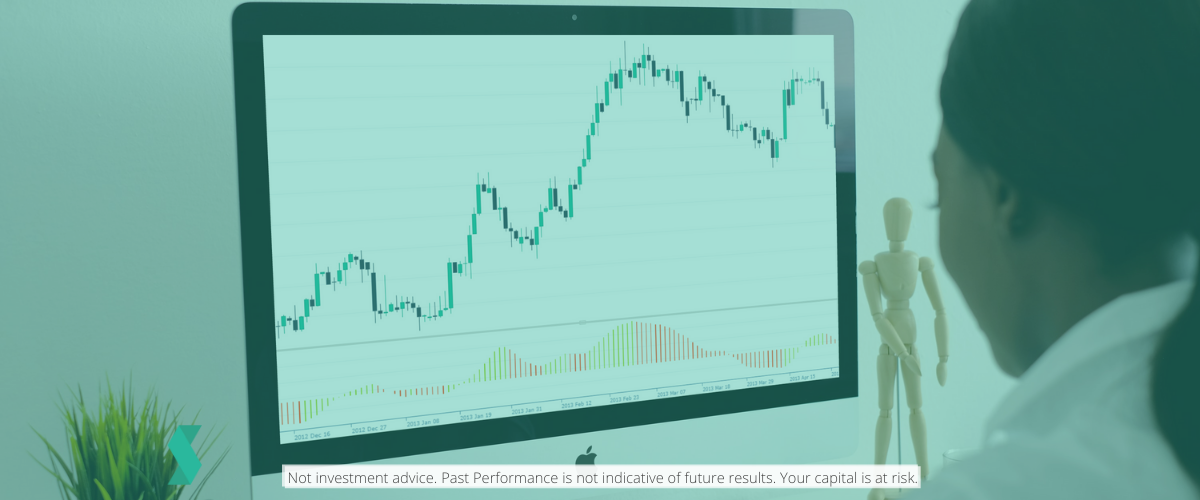# What is The Awesome Oscillator

•Skilling Writers Team
••• Education
• 交易
• Technical Analysis### The awesome oscillator

The Awesome Oscillator (AO) indicator was created by the analyst Bill Williams and is used to measure market momentum. It does this by calculating the difference between a 34 period and a five period simple moving average (SMA). Comparing two different moving averages is quite common, the twist with the Awesome Oscillator being that the moving averages are calculated using the mid-point of the bars instead of the closing point/price. Because of its nature, the Awesome Oscillator has values that fluctuate above and below a zero line. Unlike the stochastics indicator (for example), which is range bound from +100 to -100, the Awesome Oscillator is boundless.

### Red and green bars

The values created by working out the difference between a 34 period SMA and a five period SMA are plotted as a histogram of red and green bars. A bar is green when its value is higher than the previous bar. A red bar indicates that a bar is lower than the previous bar. When AO's values are above the zero line, this indicates that the short-term period is trending higher than the longer-term period. When AO's values are below the zero line, the short-term period is trending lower than the longer-term period.

### Awesome strategies

There are three potential strategies based on this indicator that the author suggested:### To go long, or buy:

‘Saucer’ - this is when the histogram bar chart is above zero, and the chart has reversed direction from a downward to upward trend. For a saucer to occur, in any three bars, the second bar is lower than the first and coloured red, and the third bar is higher than the second and coloured green.
‘Zero line crossing’ - occurs when the histogram crosses above the zero line. A consecutive run of two or more green bars is advisable as confirmation.
‘Twin peaks’ - this is when the bars turn below zero, and then there are two swing lows of the Awesome Oscillator and the second low is higher than the first. Finally the histogram bar after the second low should be green.

### Untuk pergi pendek, atau jual:

‘Saucer’ - the histogram bar chart is below zero and the chart has reversed direction from a upwards to downwards trend. A saucer occurs when, in any three bars, the second bar is higher than the first and coloured green, and the third bar is lower than the second and coloured red.
‘Zero line crossing’ - occurs when the histogram crosses below the zero line. Again, a consecutive run of two or more red bars is advisable.
‘Twin peaks’ - this is when the bars turn above zero, there are two swing highs of the awesome oscillator and the second high is lower than the first. Finally the histogram bar after the second high should be red.

Skilling summary

The Awesome Oscillator is another momentum indicator and will appeal to some as it is easy to visualise (using the histogram). In addition, the author also sets out three specific strategies that you might want to employ if using the AO. Therefore, we believe that if you like to trade using a histogram, then the Awesome Oscillator is one you could explore.